# Crack IBPS PO : Alphabet Test – Reasoning Day 71

1. How many such pair of letters are there in the word PARADIGM each of which has as many letters between them in the word as in the English alphabet?

(a) One

(b) Four

(c) Two

(d) Five

(e) None of these

e)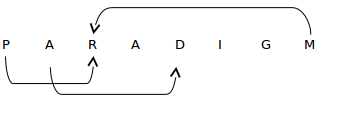2. How many such pair of letters are there in the word TUBERCULOSIS each of which has as many letters between them in the word as in the English alphabet?

(a) One

(b) Two

(c) Three

(d) Four

(e) Five

d)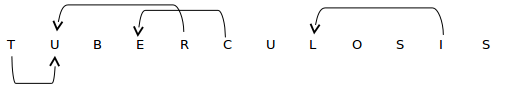TU, RU, CE, IL

3. How many such pair of letters are there in the word EXAMINER each of which has as many letters between them in the word as in the English alphabet?

(a) One

(b) Four

(c) Five

(d) Two

(e) Three

e)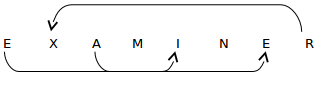4. How many such pair of letters are there in the word WOODLAND each of which has as many letters between them in the word as in the English alphabet?

(a) One

(b) Two

(c) Three

(d) Four

(e) None

b)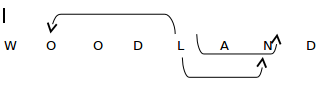LO, LN

5. How many such pair of letters are there in the word POPULATION each of which has as many letters between them in the word as in the English alphabet?

(a) Six

(b) Seven

(c) Eight

(d) Nine

(e) Ten

(e)

POPULATION

OP, OT, PT, NO, IL, IO, IP, LO, LP, OP

6. How many such pair of letters are there in the word ORGANISATION each of which has as many letters between them in the word as in the English alphabet?

(a) One

(b) Two

(c) Three

(d) Four

(e) More than Four

e)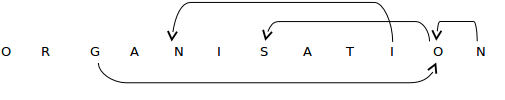GO, NO, OS, IN

7. How many such pair of letters are there in the word ELECTRIFICATION each of which has as many letters between them in the word as in the English alphabet?

(a) None

(b) Four

(c) Five

(d) Three

(e) Six

b)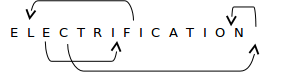EI, CN, NO, FL

8. How many such pair of letters are there in the word BEAUTIFUL each of which has as many letters between them in the word as in the English alphabet?

(a) Two

(b) Three

(c) Four

(d) Five

(e) None of these

c)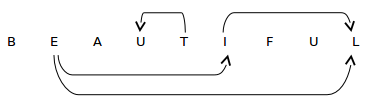EI, EL, IL, TV

9. How many such pair of letters are there in the word DISTRIBUTOR each of which has as many letters between them in the word as in the English alphabet?

(a) Four

(b) Five

(c) Six

(d) Seven

(e) Nine

(d)

D I S T R I B U T O R

DI, IR, ST, RU, RT, TU, RU

10. How many such pair of letters are there in the word DECEMBER each of which has as many letters between them in the word as in the English alphabet?

(a) One

(b) Two

(c) Three

(d) Four

(e) Five

a)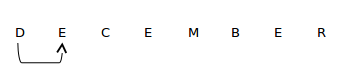DE